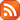Subscribe by RSS

# Math/CS Seminar

Wed., Aug. 23, 2023 2:00 p.m.

Location: CW 307.20

Speaker: Sacha Ikonicoff, University of Ottawa

Title:  Gradient descent and neural networks: a categorical approach (84 kB)Abstract:

A neural network can be thought of as a mathematical gadget which can compute a prediction from a list of inputs, using mostly parametrised linear maps. To find the right parameters and "calibrate" the neural network, one uses a technique called deep learning. Given a set of observation data, one can compute the error, i.e., the distance between the prediction given by the neural network and the observed consequence. Deep learning then uses gradient descent to update the parameters in a way which will reduce the error. This updating process is called backpropagation.

In recent years, category theorists have developed a framework to study and generalise gradient descent and neural networks. There is a construction called Para which categorises the notion of parametrised function, and a construction called Optic (a generalisation of former constructions such as Learners and Lenses), which formalises a notion of backpropagation. In this framework, neural networks are seen as maps in a small category NNet, Gradient descent is a functor from Para to Para (Optic), and deep learning can then be defined as a composition of a functor from NNet to Para with Gradient Descent.

In this talk, I will present all these notions, as well as a notion of Deep Dreaming which comes from the optic construction, with nothing as a prerequisite but first year mathematics and basic category theory!